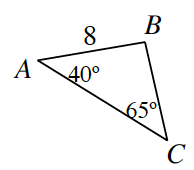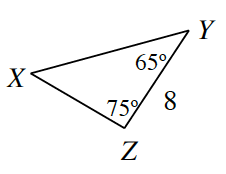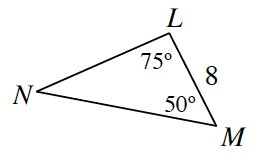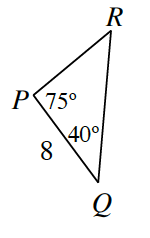Home > CCG > Chapter 6 > Lesson 6.2.1 > Problem6-58

6-58.

Decide if each triangle below is congruent to $ΔABC$ at right, similar but not congruent to $ΔABC$, or neither. Justify each answer. If you decide that they are congruent, organize your reasoning into a flowchart.1.Find the missing angles using the Triangle Angle Sum Theorem.

Similar because of $\text{AA}\sim$, but not congruent.

1.Not similar and not congruent because there are no corresponding congruent angles between the triangles.

1.